Anzeige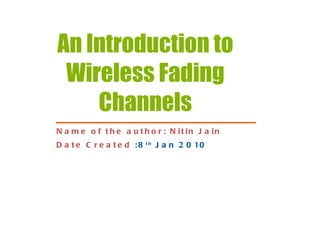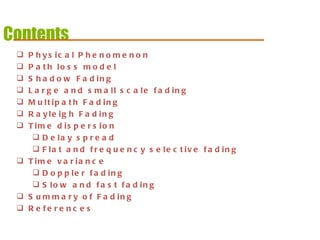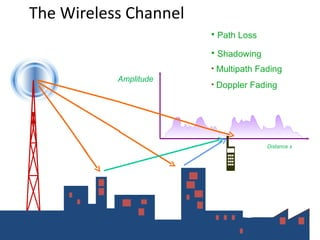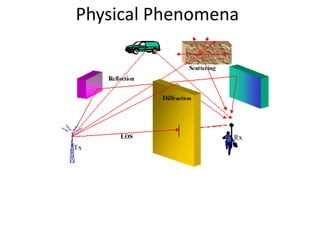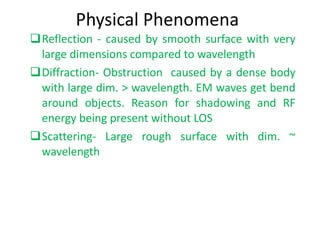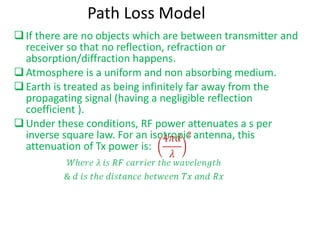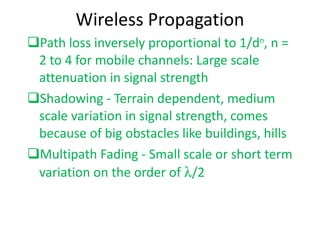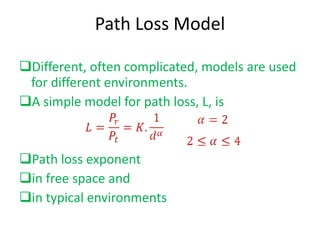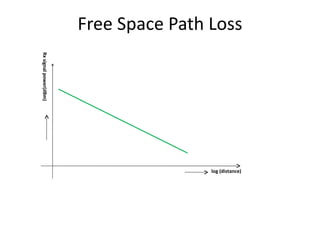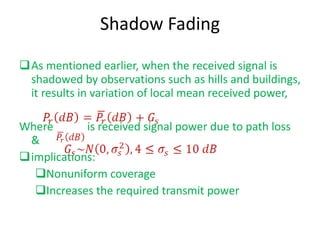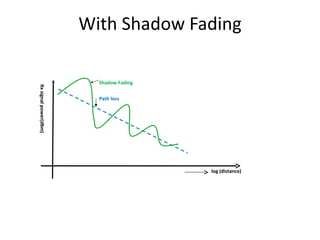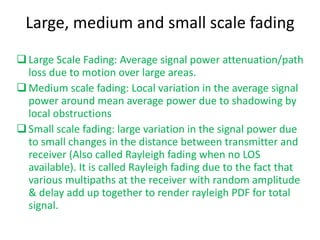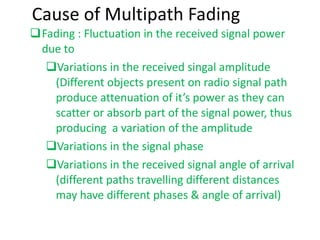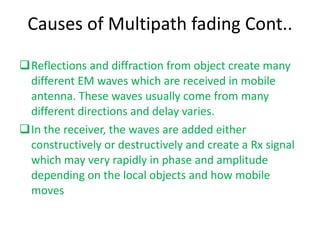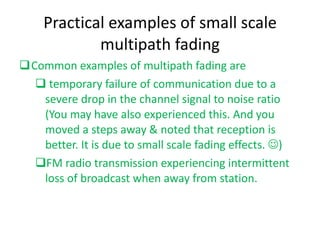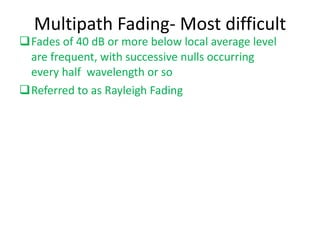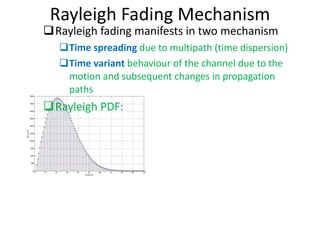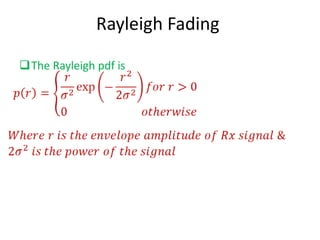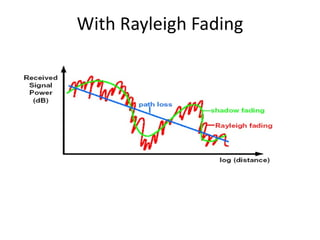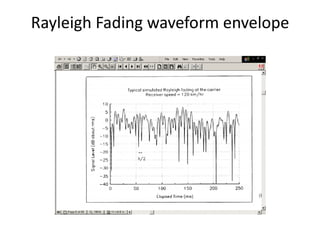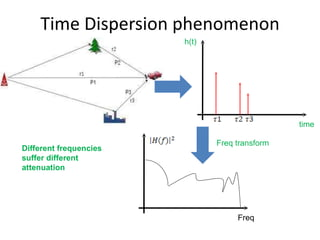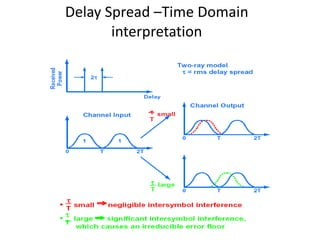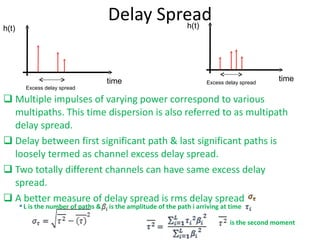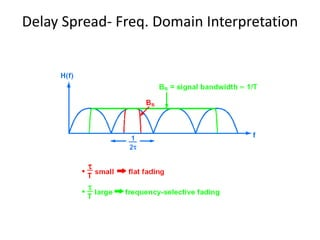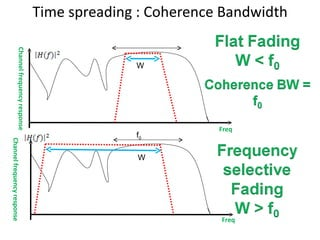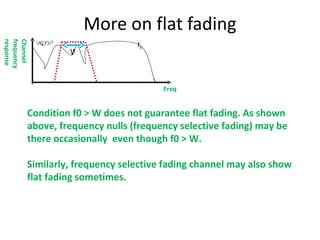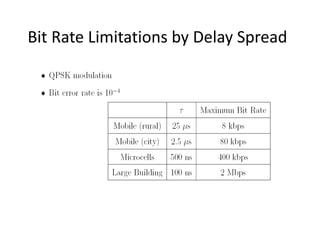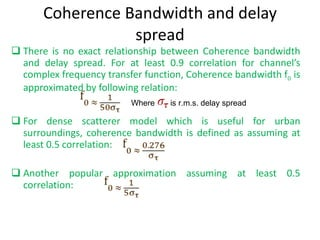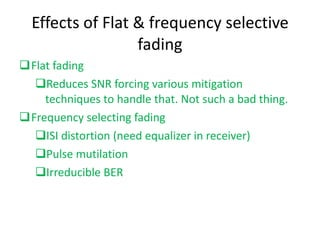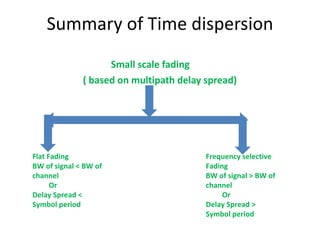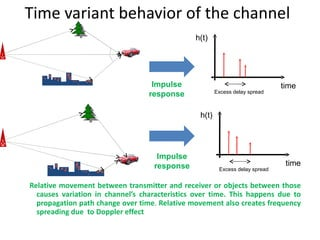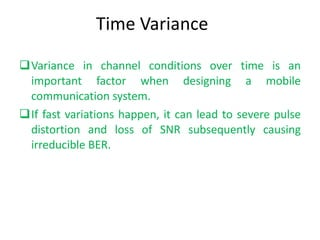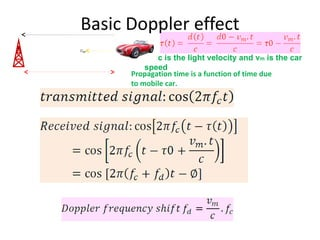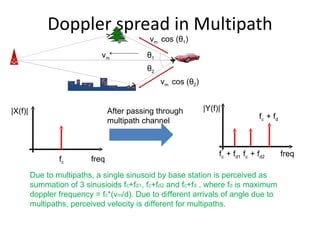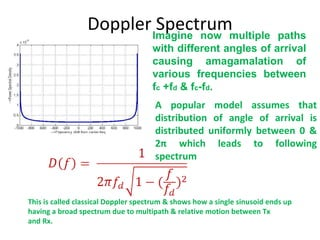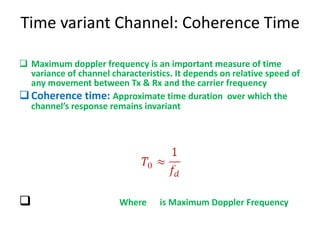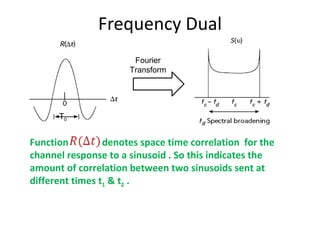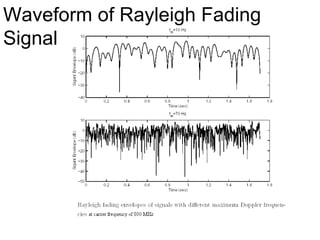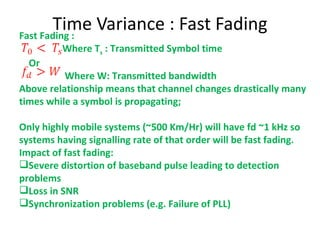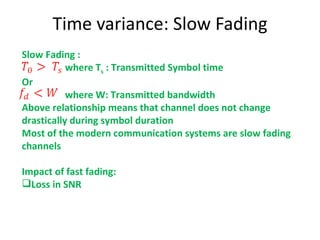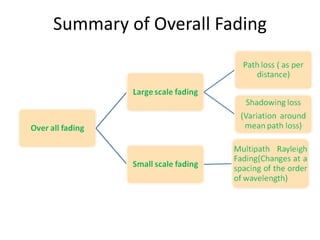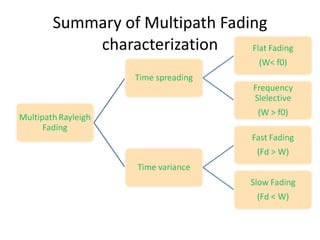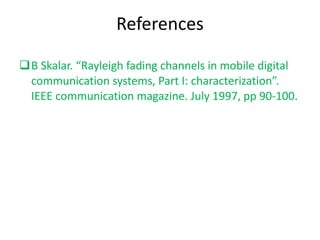1 von 43
Anzeige

### Introduction To Wireless Fading Channels

1. An Introduction to Wireless Fading Channels Name of the author: Nitin Jain Date Created : 8 th Jan 2010
2. Physical Phenomena
3. Free Space Path Loss log (distance) Rx signal power(dBm)
7. Time Dispersion phenomenon time h(t) Freq Freq transform Different frequencies suffer different attenuation
8. Delay Spread –Time Domain interpretation
9. Delay Spread- Freq. Domain Interpretation
10. Bit Rate Limitations by Delay Spread
11. Basic Doppler effect t) Propagation time is a function of time due to mobile car. c is the light velocity and v m is the car speed
12. Doppler spread in Multipath freq |X(f)| f c f c + f d1 f c + f d2 f c + f d |Y(f)| freq Due to multipaths, a single sinusoid by base station is perceived as summation of 3 sinusioids f c +f d1 , f c +f d2 and f c +f d , where f d is maximum doppler frequency = f c *(v m /d). Due to different arrivals of angle due to multipaths, perceived velocity is different for multipaths. After passing through multipath channel v m v m cos ( θ 1 ) v m cos ( θ 2 ) θ 1 θ 2
13. Doppler Spectrum This is called classical Doppler spectrum & shows how a single sinusoid ends up having a broad spectrum due to multipath & relative motion between Tx and Rx. A popular model assumes that distribution of angle of arrival is distributed uniformly between 0 & 2 π which leads to following spectrum Imagine now multiple paths with different angles of arrival causing amagamalation of various frequencies between f c +f d & f c -f d .
14. Frequency Dual Fourier Transform T 0 Function denotes space time correlation for the channel response to a sinusoid . So this indicates the amount of correlation between two sinusoids sent at different times t 1 & t 2 .
15. Waveform of Rayleigh Fading Signal